# SAT Math Multiple Choice Question 488: Answer and Explanation

### Test Information

Question: 488

8.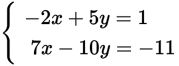If (x, y) is a solution to the system of equations above, what is the sum of x and y?

• A.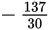• B. -4
• C.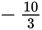• D. -3

Explanation:

B

Difficulty: Medium

Category: Heart of Algebra / Systems of Linear Equations

Strategic Advice: Because none of the variable terms has a coefficient of 1, solve the system of equations using elimination by addition (combining the equations). Before you choose an answer, check that you answered the right question (the sum of x and y).

Getting to the Answer: Multiply the top equation by 2 to eliminate the terms that have y's in them.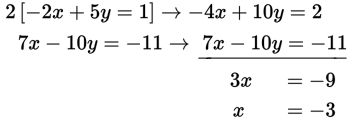Now, substitute the result into either of the original equations and simplify to find y: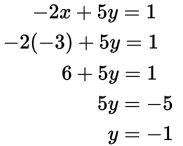The question asks for the sum, so add x and y to get -3 + (-1) = -4.